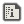# The ultimate Circle Pad tutorial.SamCreated:
Hi! This resource will (hopefully) be explaining how to do anything you need with the circle pad. Do note that the extra stick that comes with the circle pad pro and the New 3DS works the same way the regular one does.

# How to use the circle pad, the basics

The command is STICK OUT SX,SY . Or, if you want to use the Circle Pad Pro / New 3DS's extra stick, STICKEX OUT SX,SY. This command gives you the circle pad's position, SX being the horizontal position and SY being the vertical position. The circle pad's X and Y positions are a number between -0.875 and 0.875 inclusively. Note: You don't have to name them SX and SY.Here's a graphic that shows how it works. When you move the circle pad to the left, the SX variable decreases. When you move the circle pad to the right, the SX variable increases. When you move the circle pad upwards, the SY variable increases. When you move the circle pad downwards, the SY variable decreases. The green circle on the picture represents your circle pad's range.

## Example of code: Moving your character on a map

For this example, I'll be assuming that you are not using OPTION STRICT or OPTION DEFINT. If you're using STICKEX instead of STICK, don't forget to activate it beforehand with XON EXPAD!
```SPSET 0,500
WHILE 1
STICK OUT SX,SY
INC PX,SX
INC PY,-SY
SPOFS 0,PX,PY
VSYNC
WEND```
Explanation:
Spoiler
• SPSET 0,500 creates a sprite.
• WHILE 1 and WEND create a loop that goes on forever. That means everything between these two will be executed in order until you end the program.
• As I explained, STICK OUT SX,SY gives you the circle pad's position.
• INC PX,SX increases the PX variable by SX. For example, if PX is 200 and SX is 0.4, PX will now be 200.4.
• The same thing is done to PY, except for one very important thing: the SY variable is inverted!
• SPOFS 0,PX,PY moves the sprite #0 at the location defined by PX and PY.
• And VSYNC... makes everything go smoothly. (Lol, I'd explain what it exactly does but this is a basic tutorial.) Just remember to always use VSYNC in this kind of loop.

# Variables you can get out of the circle pad's position

Now we get into the advanced stuff.When you move the circle pad, as you can see, it makes two triangles which create by themselves a rectangle. Why is this relevant? Well, you can earn several variables you could need in specific contexts. Here, take a closer look.You can get the line in the middle which represents the distance between the position and the circle pad's current position. We'll call it DIST. You can also get the angle of the triangles, named A1 and A2.

## Getting the distance between the circle pad and its origin

You might need the distance between [0;0] and [SX;SY]! This could be useful, for example, in a sports game where you want to know the strength applied to the ball when you hit it.
`DIST=SQR(POW(SX,2)+POW(SY,2))`
Explanation:
SpoilerBasically, you use Pythagoras' theorem a²+b²=c². Or, with the c isolated, c=sqr(a²+b²).

## Getting the angle

You might need the angle performed by the circle pad! This could be useful, for example, in a fighting game where you want to set the angle at which your fighter's projectile will go.
```A1=DEG(ATAN(SY,SX))
A2=DEG(ATAN(SX,SY))```
Explanation:
SpoilerThis can be done with some basic trigonometry. The formula we specifically need is tanθ=opp/adj. In our situation, it becomes tanA1=SY/SX and tanA2=SX/SY. To isolate A1 and A2, you'll need to use the SmileBASIC function ATAN. Note that I convert the thing from radians (a number between 0 and π*2) to degrees (a number between 0 and 360).
Hopefully this resource will help. If there's anything I haven't explained that you would like to do with the circle pad, please ask in the comment section.Very good! I see you're trying to get that badge lol A few corrections/suggestions:
• Range is -0.875 to 0.875
• Maybe you should just add SPSET 0,0 to the example
• Explain differences between STICK and STICKEX (if there are any)I'll be coming back to this when I start creating different control schemes. Thanks!Replying to:12Me21
Very good! I see you're trying to get that badge lol A few corrections/suggestions:
• Range is -0.875 to 0.875
• Maybe you should just add SPSET 0,0 to the example
• Explain differences between STICK and STICKEX (if there are any)
Done!Dang, looks really good! Thank you!I've Managed to turn the Circle pad X,Y into a 360 degree angle
```STICK OUT SX, SY
R=DEG(ATAN(-SY, SX))```